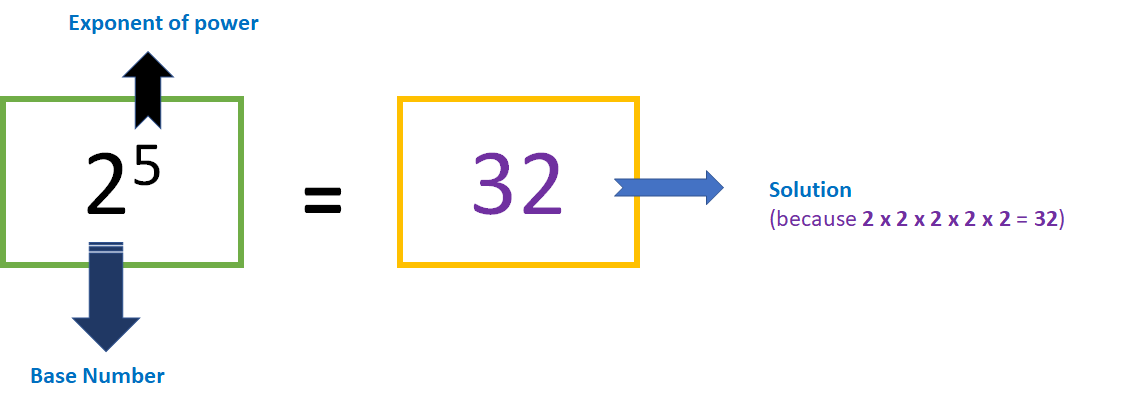Select Page

# Calculate Power of Number in ABAP

by | Jun 3, 2018 | ABAP Programs

Home » SAP » ABAP » ABAP Programs » Calculate Power of Number in ABAP

Preface – This post is part of the ABAP Programs series.

Sometimes, there is a need to know the power of a number provided by user. In that case we program to calculate power of number in ABAP. It is not a question that is merely asked in an interview but an important keyword that is used in day to day programming.

## Introduction

To calculate power of a number, you need to multiply that number by itself n times. Here n is the power. To achieve this thing we need to use Loops in ABAP. Following program implements the same:Power of Number in ABAP- Illustration Image

## ABAP Program

```PARAMETERS: lv_data1(10) type i,
lv_data2(10) type i.
While  lv_data2 > 1 .
lv_data1 = lv_data1 * lv_data1.
lv_data2 = lv_data2 - 1.
ENDWHILE.
Write: lv_data1.
```

## Explanation

In the program, mentioned above, we have implemented a while loop to get the required result. The same is explained below, step by step:

1. Initially, we have defined two parameters: lv_data1 and lv_data2. Both of these parameters are of type i.e. integer and length 10. These parameters will be used to take the number (also called base number) and the power ( also called exponent of power).
2. Then, we will use a while loop which will run from the power value to 1.
3. We will keep multiplying the number with itself till the loop runs.
4. In this line, we will keep decreasing the value of power till it is equal to 1.
5. Now, we have calculated the power of the number. The output will be then printed using ABAP keyword “WRITE”.

## Author

•A Full Stack Developer with 10+ years of experience in technical content creation.

•